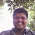## Thursday, October 27, 2011

### IIT-JEE 2011 and IIT-JEE 2010 Questions on Doppler Effect

"I am a friend of Plato, I am a friend of Aristotle, but truth is my greater friend."
– Sir Isaac Newton

Questions on Doppler Effect were discussed on this site earlier. You can access them by clicking on the label ‘Doppler effect’ below this post or by trying a search for ‘Doppler effect’ using the search box provided on this page. Questions on Doppler effect appear frequently in Entrance examination question papers. Today we will discuss two questions in this section Here is the first question which appeared in IIT-JEE 2011 question paper as a single answer type multiple choice question:

(1) A police car with a siren of frequency 8 kHz is moving with uniform velocity 36 km/hr towards a tall building which reflects the sound waves. The speed of sound in air is 320 m/s. The frequency of the siren heard by the car driver is

(A) 8.50 kHz

(B) 8.25 kHz

(C) 7.75 kHz

(D) 7.50 kH

The general expression for the apparent frequency (n’) produced due to Doppler effect is

n’ = n(v+w–vL)/(v+w–vS) where ‘v’ is the velocity of sound, ‘w’ is the wind velocity ‘vL’ is the velocity of the listener, ‘vS’ is the velocity of the source of sound and ‘n’ is the actual frequency of the sound emitted by the source. Note that all the velocities in this expression are in the same direction and the source is behind the listener. In other words, the listener is moving away from the source and the source is moving towards the listener.

[It will be helpful to remember that if the source moves towards the listener or the listener moves towards the source, the apparent frequency increases. If they move away, the apparent frequency decreases].

In the present case the source of sound which produces the Doppler effect is the reflected image of the siren. (The car driver will not detect any change in the frequency of the direct sound from the siren since he is moving along with the siren). The reflected image (source) of the siren moves towards the car driver with a speed of 36 km/hr and the car driver (listener) moves towards the reflected image (source) with the same speed.

Thus in the expression for apparent frequency we have ro substitute

n = 8 kHz, vL = 36 km/hr = 36×(5/18) ms–1 = –10 ms–1, w = 0, vS = + 10 ms–1.

Therefore, n’ = 8×(320 + 10)/(320 – 10) = 8×(33/31) kHz = 8.5 kHz.

The following question appeared in IIT-JEE 2010 question paper as an integer type question (in which the answer is a single-digit integer, ranging from 0 to 9):

(2) A stationary source is emitting sound at a fixed frequency f0, which is reflected by two cars approaching the source. The difference between the frequencies of sound reflected from the cars is 1.2% of f0. What is the difference in the speeds of the cars (in km per hour) to the nearest integer? The cars are moving at constant speeds much smaller than the speed of sound which is 330 ms–1.

This is similar to question no.1 above. The difference between the frquencies of the sound reflected from the cars is given by

n1 n2’ = [f0(v+v1)/(v–v1)] – [f0(v+v2)/(v–v2)] where v1 and v2 are the speeds of the two cars.

Therefore (n1 n2’)/f0 = [(v+v1)/(v–v1)] – [(v+v2)/(v–v2)]

The quantity on the left hand side of the above equation is 1.2/100 as given in the question.

Therefore, 0.012 = [(v+v1) (v–v2) (v+v2) (v–v1)] / [(v–v1)(v–v2)]

Or, 0.012 = (2 vv1 2 vv2)/ [(v–v1)(v–v2)] = 2v(v1 –v2)/v2 since (v–v1) (v–v2) ≈ v

[The cars are moving at speeds much smaller than the speed of sound].

Therefore we have

0.012 = 2(v1 –v2)/v from which (v1 –v2) = 0.006×330 ms–1

The difference in the speeds of the cars in km per hour is 0.006×330×(18/5) = 7.128

The answer, which is the nearest integer, is 7.

#### 3 comments:

1.dude it is the best site for a proper learning

2.In 2nd question if we take the assumption before solving the answer becomes double. Why?????

3.You cannot make the assumption before solving since the correct difference in speeds of the cars is contained in the terms which you try to approximate by the assumption